Updating search results...

# 23 Results

View
Selected filters:
• meanEducational Use
Rating
0.0 stars

In this video segment from TV411, figure skaters compute their average daily practice time.

Subject:
Mathematics
Material Type:
Lecture
Provider:
PBS LearningMedia
Author:
U.S. Department of Education
WNET
07/10/2008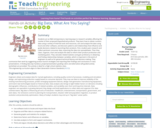Educational Use
Rating
0.0 stars

Students act as R&D entrepreneurs, learning ways to research variables affecting the market of their proposed (hypothetical) products. They learn how to obtain numeric data using a variety of Internet tools and resources, sort and analyze the data using Excel and other software, and discover patterns and relationships that influence and guide decisions related to launching their products. First, student pairs research and collect pertinent consumer data, importing the data into spreadsheets. Then they clean, organize, chart and analyze the data to inform their product production and marketing plans. They calculate related statistics and gain proficiency in obtaining and finding relationships between variables, which is important in the work of engineers as well as for general technical literacy and decision-making. They summarize their work by suggesting product launch strategies and reporting their findings and conclusions in class presentations. A finding data tips handout, project/presentation grading rubric and alternative self-guided activity worksheet are provided. This activity is ideal for a high school statistics class.

Subject:
Mathematics
Statistics and Probability
Material Type:
Activity/Lab
Provider:
TeachEngineering
Provider Set:
Activities
Author:
Tom Falcone
05/03/2017Educational Use
Rating
0.0 stars

Students learn a simple technique for quantifying the amount of photosynthesis that occurs in a given period of time, using a common water plant (Elodea). They can use this technique to compare the amounts of photosynthesis that occur under conditions of low and high light levels. Before they begin the experiment, however, students must come up with a well-worded hypothesis to be tested. After running the experiment, students pool their data to get a large sample size, determine the measures of central tendency of the class data, and then graph and interpret the results.

Subject:
Engineering
Science
Material Type:
Activity/Lab
Lesson Plan
Provider:
TeachEngineering
Provider Set:
TeachEngineering
Author:
Mary R. Hebrank
09/26/2008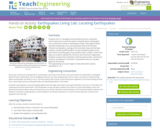Educational Use
Rating
0.0 stars

Students use U.S. Geological Survey (USGS) real-time, real-world seismic data from around the planet to identify where earthquakes occur and look for trends in earthquake activity. They explore where and why earthquakes occur, learning about faults and how they influence earthquakes. Looking at the interactive maps and the data, students use Microsoft® Excel® to conduct detailed analysis of the most-recent 25 earthquakes; they calculate mean, median, mode of the data set, as well as identify the minimum and maximum magnitudes. Students compare their predictions with the physical data, and look for trends to and patterns in the data. A worksheet serves as a student guide for the activity.

Subject:
Earth and Space Science
Science
Material Type:
Activity/Lab
Provider:
TeachEngineering
Provider Set:
TeachEngineering
Author:
Jessica Noffsinger
Jonathan Knudtsen
Karen Johnson
Mike Mooney
Minal Parekh
Scott Schankweiler
02/17/2021Educational Use
Rating
0.0 stars

Students explore the concept of the mean as they examine and compare scores for various sports events.

Subject:
Mathematics
Material Type:
Lesson Plan
Provider:
PBS LearningMedia
Author:
U.S. Department of Education
WNET
07/25/2008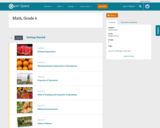Conditional Remix & Share Permitted
CC BY-NC
Rating
0.0 stars
Rating
0.0 stars
Subject:
Mathematics
Provider:
Pearson
02/28/2022Conditional Remix & Share Permitted
CC BY-NC
Rating
0.0 stars

Distributions and Variability

Type of Unit: Project

Prior Knowledge

Students should be able to:

Represent and interpret data using a line plot.
Understand other visual representations of data.

Lesson Flow

Students begin the unit by discussing what constitutes a statistical question. In order to answer statistical questions, data must be gathered in a consistent and accurate manner and then analyzed using appropriate tools.

Students learn different tools for analyzing data, including:

Measures of center: mean (average), median, mode
Measures of spread: mean absolute deviation, lower and upper extremes, lower and upper quartile, interquartile range
Visual representations: line plot, box plot, histogram

These tools are compared and contrasted to better understand the benefits and limitations of each. Analyzing different data sets using these tools will develop an understanding for which ones are the most appropriate to interpret the given data.

To demonstrate their understanding of the concepts, students will work on a project for the duration of the unit. The project will involve identifying an appropriate statistical question, collecting data, analyzing data, and presenting the results. It will serve as the final assessment.

Subject:
Mathematics
Statistics and Probability
Provider:
PearsonConditional Remix & Share Permitted
CC BY-NC
Rating
0.0 stars

Groups begin presentations for their unit project. Students provide constructive feedback on others' presentations.Key ConceptsThe unit project serves as the final assessment. Students should demonstrate their understanding of unit concepts:Measures of center (mean, median, mode) and spread (MAD, range, interquartile range)The five-number summary and its relationship to box plotsRelationship between data sets and line plots, box plots, and histogramsAdvantages and disadvantages of portraying data in line plots, box plots, and histogramsGoals and Learning ObjectivesPresent projects and demonstrate an understanding of the unit concepts.Provide feedback for others' presentations.Review the concepts from the unit.

Subject:
Statistics and Probability
Material Type:
Lesson Plan
Author:
02/28/2022Conditional Remix & Share Permitted
CC BY-NC
Rating
0.0 stars

Remaining groups present their unit projects. Students discuss teacher and peer feedback.Key ConceptsThe unit project serves as the final assessment. Students should demonstrate their understanding of unit concepts:Measures of center (mean, median, mode) and spread (MAD, range, interquartile range)The five-number summary and its relationship to box plotsRelationship between data sets and line plots, box plots, and histogramsAdvantages and disadvantages of portraying data in line plots, box plots, and histogramsGoals and Learning ObjectivesPresent projects and demonstrate an understanding of the unit concepts.Provide feedback for others' presentations.Review the concepts from the unit.Review presentation feedback and reflect.

Subject:
Statistics and Probability
Material Type:
Lesson Plan
Author:
02/28/2022Conditional Remix & Share Permitted
CC BY-NC
Rating
0.0 stars

In this lesson, students draw a line plot of a set of data and then find the mean of the data. This lesson also informally introduces the concepts of the median, or middle value, and the mode, or most common value. These terms will be formally defined in Lesson 6.Using a sample set of data, students review construction of a line plot. The mean as fair share is introduced as well as the algorithm for mean. Using the sample set of data, students determine the mean and informally describe the set of data, looking at measures of center and the shape of the data. Students also determine the middle 50% of the data.Key ConceptsThe mean is a measure of center and is one of the ways to determine what is typical for a set of data.The mean is often called the average. It is found by adding all values together and then dividing by the number of values.A line plot is a visual representation of the data. It can be used to find the mean by adjusting the data points to one value, such that the sum of the data does not change.Goals and Learning ObjectivesReview construction of a line plot.Introduce the concept of the mean as a measure of center.Use the fair-share method and standard algorithm to find the mean.

Subject:
Statistics and Probability
Material Type:
Lesson Plan
Author:
02/28/2022Conditional Remix & Share Permitted
CC BY-NC
Rating
0.0 stars

Subject:
Statistics and Probability
Material Type:
Lesson Plan
Author:
02/28/2022Conditional Remix & Share Permitted
CC BY-NC
Rating
0.0 stars

GalleryCreate a Data SetStudents will create data sets with a specified mean, median, range, and number of data values.Bouncing Ball Experiment How high does the class think a typical ball bounces (compared to its drop height) on its first bounce? Students will conduct an experiment to find out.Adding New Data to a Data Set Given a data set, students will explore how the mean changes as they add data values.Bowling Scores Students will create bowling score data sets that meet certain criteria with regard to measures of center.Mean Number of Fillings Ten people sit in a dentist's waiting room. The mean number of fillings they have in their teeth is 4, yet none of them actually have 4 fillings. Students will explain how this situation is possible.Forestland Students will examine and interpret box plots that show the percentage of forestland in 20 European countries.What's My Data?Students will create a data set that fits a given histogram and then adjust the data set to fit additional criteria.What's My Data 2? Students will create a data set that fits a given box plot and then adjust the data set to fit additional criteria.Compare Graphs Students will make a box plot and a histogram that are based on a given line plot and then compare the three graphs to decide which one best represents the data.Random Numbers What would a data set of randomly generated numbers look like when represented on a histogram? Students will find out!No Telephone? The U.S. Census Bureau provides state-by-state data about the number of households that do not have telephones. Students will examine two box plots that show census data from 1960 and 1990 and compare and analyze the data.Who Is Taller?Who is taller—the boys in the class or the girls in the class? Students will find out by separating the class height data gathered earlier into data for boys and data for girls.

Subject:
Statistics and Probability
Material Type:
Lesson Plan
Author:
02/28/2022Conditional Remix & Share Permitted
CC BY-NC
Rating
0.0 stars

In this lesson, students are given criteria about measures of center, and they must create line plots for data that meet the criteria. Students also explore the effect on the median and the mean when values are added to a data set.Students use a tool that shows a line plot where measures of center are shown. Students manipulate the graph and observe how the measures are affected. Students explore how well each measure describes the data and discover that the mean is affected more by extreme values than the mode or median. The mathematical definitions for measures of center and spread are formalized.Key ConceptsStudents use the Line Plot with Stats interactive to develop a greater understanding of the measures of center. Here are a few of the things students may discover:The mean and the median do not have to be data points.The mean is affected by extreme values, while the median is not.Adding values above the mean increases the mean. Adding values below the mean decreases the mean.You can add values above and below the mean without changing the mean, as long as those points are “balanced.”Adding values above the median may or may not increase the median. Adding values below the median may or may not decrease the median.Adding equal numbers of points above and below the median does not change the median.The measures of center can be related in any number of ways. For example, the mean can be greater than the median, the median can be greater than the mean, and the mode can be greater than or less than either of these measures.Note: In other courses, students will learn that a set of data may have more than one mode. That will not be the case in this lesson.Goals and Learning ObjectivesExplore how changing the data in a line plot affects the measures of center (mean, median).Understand that the mean is affected by outliers more than the median is.Create line plots that fit criteria for given measures of center.

Subject:
Statistics and Probability
Material Type:
Lesson Plan
Author:
02/28/2022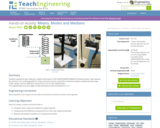Educational Use
Rating
0.0 stars

Students experience data collection, analysis and inquiry in this LEGO® MINDSTORMS® NXT -based activity. They measure the position of an oscillating platform using a ultrasonic sensor and perform statistical analysis to determine the mean, mode, median, percent difference and percent error for the collected data.

Subject:
Engineering
Mathematics
Physics
Science
Material Type:
Activity/Lab
Provider:
TeachEngineering
Provider Set:
TeachEngineering
Author:
Irina Igel
Noam Pillischer
Ronald Poveda
09/18/2014Educational Use
Rating
0.0 stars

Students learn about the statistical analysis of measurements and error propagation, reviewing concepts of precision, accuracy and error types. This is done through calculations related to the concept of density. Students work in teams to each measure the dimensions and mass of five identical cubes, compile the measurements into small data sets, calculate statistics including the mean and standard deviation of these measurements, and use the mean values of the measurements to calculate density of the cubes. Then they use this calculated density to determine the mass of a new object made of the same material. This is done by measuring the appropriate dimensions of the new object, calculating its volume, and then calculating its mass using the density value. Next, the mass of the new object is measured by each student group and the standard deviation of the measurements is calculated. Finally, students determine the accuracy of the calculated mass by comparing it to the measured mass, determining whether the difference in the measurements is more or less than the standard deviation.

Subject:
Engineering
Mathematics
Measurement and Data
Science
Material Type:
Activity/Lab
Provider:
TeachEngineering
Provider Set:
TeachEngineering
Author:
Ralph Cox
10/14/2015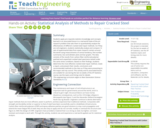Educational Use
Rating
0.0 stars

Students apply pre-requisite statistics knowledge and concepts learned in an associated lesson to a real-world state-of-the-art research problem that asks them to quantitatively analyze the effectiveness of different cracked steel repair methods. As if they are civil engineers, students statistically analyze and compare 12 sets of experimental data from seven research centers around the world using measurements of central tendency, five-number summaries, box-and-whisker plots and bar graphs. The data consists of the results from carbon-fiber-reinforced polymer patched and unpatched cracked steel specimens tested under the same stress conditions. Based on their findings, students determine the most effective cracked steel repair method, create a report, and present their results, conclusions and recommended methods to the class as if they were presenting to the mayor and city council. This activity and its associated lesson are suitable for use during the last six weeks of the AP Statistics course; see the topics and timing note for details.

Subject:
Mathematics
Statistics and Probability
Material Type:
Activity/Lab
Provider:
TeachEngineering
Provider Set:
TeachEngineering
Author:
Botong Zheng
Miguel R. Ramirez
Mina Dawood
02/17/2021Educational Use
Rating
0.0 stars

Working as if they are engineers aiming to analyze and then improve data collection devices for precision agriculture, students determine how accurate temperature sensors are by comparing them to each other. Teams record soil temperature data during a class period while making changes to the samples to mimic real-world crop conditions—such as the addition of water and heat and the removal of the heat. Groups analyze their collected data by finding the mean, median, mode, and standard deviation. Then, the class combines all the team data points in order to compare data collected from numerous devices and analyze the accuracy of their recording devices by finding the standard deviation of temperature readings at each minute. By averaging the standard deviations of each minute’s temperature reading, students determine the accuracy of their temperature sensors. Students present their findings and conclusions, including making recommendations for temperature sensor improvements.

Subject:
Mathematics
Statistics and Probability
Material Type:
Activity/Lab
Provider:
TeachEngineering
Provider Set:
Activities
Author:
Keith Lehman
Northern Cass
Trent Kosel
06/28/2017Conditional Remix & Share Permitted
CC BY-NC-SA
Rating
0.0 stars

This 7-minute video lesson gives formulas for the Bernoulli Distribution Mean and Variance. [Statistics playlist: Lesson 42 of 85]

Subject:
Mathematics
Statistics and Probability
Material Type:
Lecture
Provider:
Provider Set:
Author:
Salman Khan
02/20/2011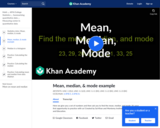Conditional Remix & Share Permitted
CC BY-NC-SA
Rating
0.0 stars

This 4-minute video lesson explains the mean, median, and mode. [Statistics playlist: Lesson 1 of 85]

Subject:
Mathematics
Statistics and Probability
Material Type:
Lecture
Provider:
Provider Set:
Author:
Salman Khan
02/20/2011Conditional Remix & Share Permitted
CC BY-NC-SA
Rating
0.0 stars

This 8-minute video lesson gives an example of the mean and variance of Bernoulli Distribution. [Statistics playlist: Lesson 41 of 85]

Subject:
Mathematics
Statistics and Probability
Material Type:
Lecture
Provider: